Conservation Of Energy

CONSERVATION OF ENERGY THEOREM

Nothing can be destroyed or created in the universe like energy. Suppose that a ball falls from height of 2m, it has only potential energy at the beginning, however, as it falls it gains kinetic energy and its velocity increases. When it hits the ground it has only kinetic energy. Well, where is the potential energy that it has at the beginning? It is totally converted to the kinetic energy, as said in the first sentence nothing can be destroyed or created they just change form. Thus, our potential energy also changes its forms from potential to the kinetic energy. In summary, energy of the system is always constant, they can change their forms but amount of total energy does not change.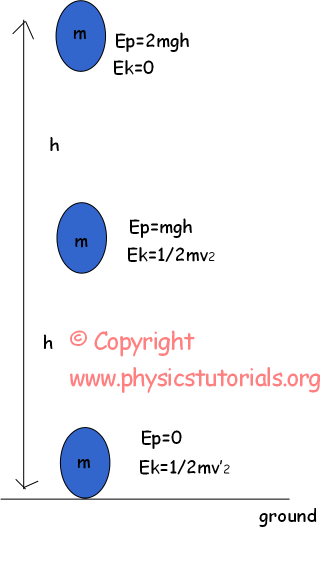Picture shows the energy change of the ball. It has only potential energy 2mgh at the beginning. When it starts to lose height it gains velocity in other word decreasing in the amount of potential energy increases the amount of kinetic energy. At h height it has both potential and kinetic energy and when it hits the ground the potential energy becomes zero and kinetic energy has its maximum value.

Einitial = Efinal

Example: By using the given information in picture given below, find the velocity of the ball at point D.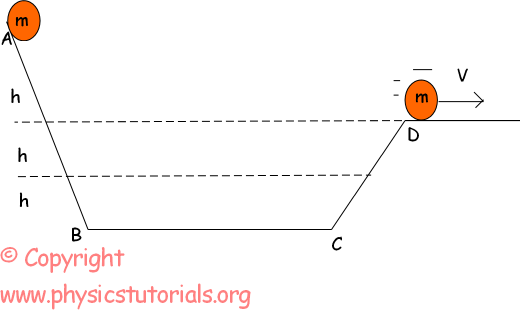Ei=Ef (conservation of energy)

mg3h=mg2h+1/2mv²

mgh=1/2mv²

v=√2gh

Example: A block having mass 2kg and velocity 2m/s slide on the inclined plane. If the horizontal surface has friction constant µ=0, 4 find the distance it travels in horizontal before it stops.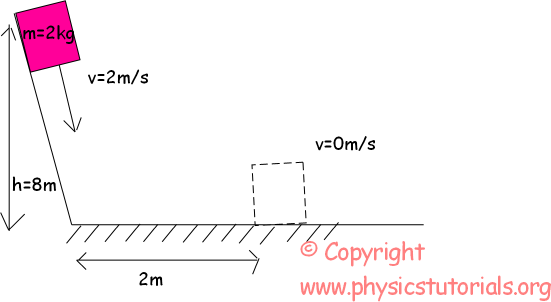We use conservation of energy in solution of this problem.

Einitial=Efinal

Einitial=Ep+Ek=mgh+1/2mv² Efinal=0

Einitial=2kg.10m/s².8m+1/2.2kg. (2m/s) ² Work done by friction=Einitial Einitial=164joule

Wfriction=µ.N.X=0,4.2kg.10m/s².X=Ei

8. X=164joule X=20,5m

Block slides 20,5m in horizontal

Example: Find the final velocity of the box from the given picture.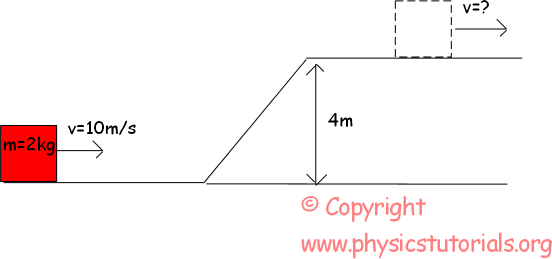We again use the conservation of energy theorem.

Einitial must be equal to the Efinal.

Einitial=Ek=1/2mv² Efinal=Ek+Ep=1/2mv’²+mgh

Ei=1/2.2kg.(10m/s) ²=100joule Efinal=1/2.2kg.v’²+2kg.10m/s².4m=80+v’²

100=80+v’² v’=25m/s

Example: Find the amount of compression of the spring if the ball does free fall from 4m and compresses the spring.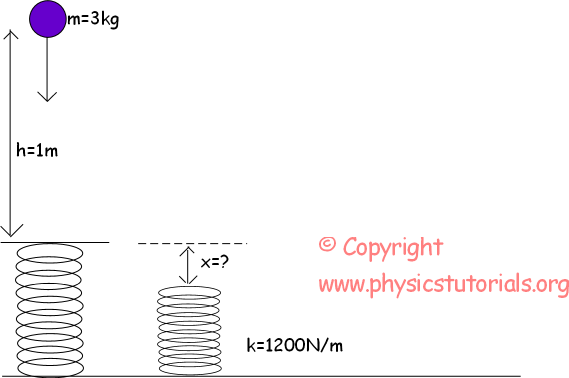From the conservation of energy law we can find the amount of spring’s compression.

Ep=1/2.kx² for spring

X=1/4m

Ball compresses the spring 1/4m.

Author: